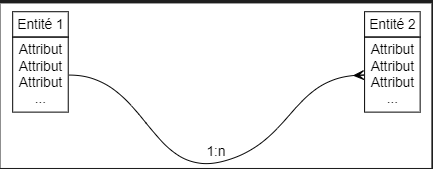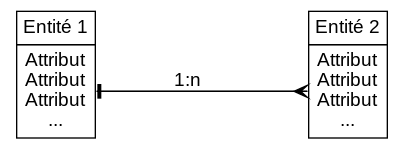# Arrowtail and arrowhead with same rank?

Arrowhead and arrowtail work fine when I make a LR rank :

``````nodesep = 2;                /* increase distance between nodes */
{ rank = lr; set1 set2 }
set1:1 -> set2:1 [dir=both, arrowtail=tee, arrowhead=crow label="1:n" fontname = "Arial"];
}
``````

How can I have arrowtail and arrowhead with same rank ? It doesnt work for me…

``````nodesep = 2;                /* increase distance between nodes */
{ rank = same; set1 set2 }
set1:1 -> set2:1 [dir=both, arrowtail=tee, arrowhead=crow label="1:n" fontname = "Arial"];
}
``````Please include a complete input file (reduced to a minimum that causes the problem, if possible). In particular, it is important to see how set1 & set2 are defined.
Also, please include any error and warning messages from dot

Here is the full code. when I change `rank=same` to `rank=LR` eveything works fine…but it’s not what I want. (I want the tables side by side). There is no error.

``````digraph G
{
node[ shape = none, fontname = "Arial" fontsize =14 ];

set1[ label=<
<TR>
<TD colspan="1"   align="center">Entité 1</TD>
</TR>
<TR>
<TD PORT="1">Attribut<BR/>Attribut<BR/>Attribut<BR/>...</TD>
</TR>

</TABLE>>];

set2[ label=<
<TR>
<TD colspan="1"   align="center">Entité 2</TD>
</TR>
<TR>
<TD PORT="1">Attribut<BR/>Attribut<BR/>Attribut<BR/>...</TD>
</TR>

</TABLE>>];

# layout
nodesep = 2;                /* increase distance between nodes */
{ rank = same; set1 set2 }
set1:1 -> set2:1 [dir=both arrowtail=tee arrowhead=crow label="1:n" fontname = "Arial"];
``````

}

Unfortunately, you have encountered a known bug (When using HTML table and port, arrows are sometimes misplaced (#1357) · Issues · graphviz / graphviz · GitLab).
A fairly painless(?) work-around is to replace the single two-headed edge with two single-direction edges, like so:

``````digraph G
{
node[ shape = none, fontname = "Arial" fontsize =14 ];

set1[ label=<
<TR>
<TD colspan="1"   align="center">Entité 1</TD>
</TR>
<TR>
<TD PORT="1">Attribut<BR/>Attribut<BR/>Attribut<BR/>...</TD>
</TR>
</TABLE>>];

set2[ label=<
<TR>
<TD colspan="1"   align="center">Entité 2</TD>
</TR>
<TR>
<TD PORT="1">Attribut<BR/>Attribut<BR/>Attribut<BR/>...</TD>
</TR>
</TABLE>>];

# layout
nodesep = 2;                /* increase distance between nodes */
{ rank = same; set1 set2 }
//set1:1 -> set2:1 [Xdir=both arrowtail=tee arrowhead=crow xlabel="1:n" fontname = "Arial"];
// replace two-headed edge with two simpler edges
set1:1 -> set2:1 [ arrowhead=crow xlabel="1:n" fontname = "Arial"];
set2:1 -> set1:1 [ arrowhead=tee ]
}
``````

Giving: